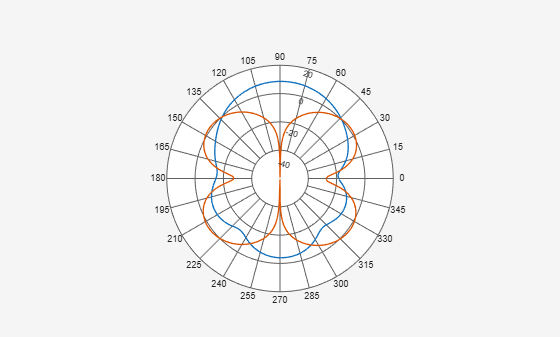# findLobes

Main, back, and side lobe data

## Syntax

``L = findLobes(p)``
``L = findLobes(p,index)``

## Description

example

````L = findLobes(p)` returns a structure, `L`, defining the main, back, and side lobes of the antenna or array radiation pattern in the specified polar plot, `p`.```

example

````L = findLobes(p,index)` returns the radiation pattern lobes from the data set specified in `index`.```

## Input Arguments

expand all

Polar plot, specified as a scalar handle.

Index of data set, specified as a scalar.

## Output Arguments

expand all

Main,back, and side lobe data, returned as a structure.

## Examples

expand all

Create a dipole antenna and calculate its directivity at 270 MHz.

```d = dipole; D = pattern(d,270e6,0,0:1:360);```

Create a polar plot of the dipole directivity. Find the main, back, and side lobes of the dipole antenna.

`p = polarpattern(D);``L = findLobes(p)`
```L = struct with fields: mainLobe: [1x1 struct] backLobe: [1x1 struct] sideLobes: [1x1 struct] FB: 0.0129 SLL: 0 HPBW: 30.9141 FNBW: 89.7507 FBIdx: [216 35.5000] SLLIdx: [216 326] HPBWIdx: [202 233] HPBWAng: [200.4432 231.3573] FNBWIdx: [181 271] ```

Inspect main, back, and side lobe data.

`MainLobe = L.mainLobe`
```MainLobe = struct with fields: index: 216 magnitude: 3.6685 angle: 214.4044 extent: [181 271] ```
`BackLobe = L.backLobe`
```BackLobe = struct with fields: magnitude: 3.6556 angle: 34.4044 extent: [361 91] index: 35.5000 ```
`SideLobe = L.sideLobes`
```SideLobe = struct with fields: index: 326 magnitude: 3.6685 angle: 324.0997 extent: [2x2 double] ```

Create a helix antenna that has a 28 mm radius, a 1.2 mm width, and 4 turns. Calculate and plot the directivity of the antenna at 1.8 GHz.

```hx = helix('Radius',28e-3,'Width',1.2e-3,'Turns',4); H = pattern(hx, 1.8e9,0,0:1:360); P = polarpattern(H);```Create a dipole antenna and calculate the directivity at 270 MHz.

```d = dipole; D = pattern(d,270e6,0,0:1:360);```

Add the directivity of the dipole to the existing polar plot.

`add(P,D);`Find the main, back, and side lobes of helix antenna.

`L = findLobes(P,1)`
```L = struct with fields: mainLobe: [1x1 struct] backLobe: [1x1 struct] sideLobes: [1x1 struct] FB: 11.1683 SLL: 11.1166 HPBW: 56.8421 FNBW: 172.5208 FBIdx: [90 270.5000] SLLIdx: [90 273] HPBWIdx: [61 118] HPBWAng: [59.8338 116.6759] FNBWIdx: [4 177] ```

## Version History

Introduced in R2016a